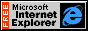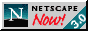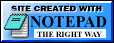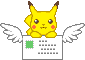Full Stack and Embedded Developer

# How to interpolate wind data

This article is a work in progress and will be revised several times. This is a loose series of words and code blocks to put my thoughts into words to help myself better understand the work I am doing. Please note that Metal is a c++-like language and is missing many core features of C++11 such as `new` & `delete` operators, `memalloc`, `memset`, `free`, inheritance/derived classes, the foundational `stdlib` (though it comes with it’s own stripped down `metal_stdlib` with apple branded alternatives such as `vec<T, n>` instead of `std::vector<T>`), etc.

This is meant to be a reference article for non-scientific projects. This article has been heavily simplified for performance and “close enough” approximations.

## Interpolation

If you’re here reading this, you probably know that interpolation is used when you have a series of data, either through time or space, and you want to estimate the data between your data points. If X₀=0, Y₀=0 and X₂=2, Y₂=4, then X₁=1 means Y₁ probably equals 2. We can guess this because we can see the 2 values make up a line similar to Y=2X, or maybe it’s Y=X^2. Who’s to say? What’s important is using the data we have around us to best-guess this.

## Problem & Requirements before Interpolation

Map Distortion and Scaling were unique to our project, however they may interest you in similar projects. These are not required for interpolation at all. These are for if you were planning on putting a view with an X/Y coordinate system on a map and these problems weren’t handled for you.

### Map Distortion

Our data was given to us in a 2D space with an XY coordinate system, however it was not processed for distortion. To do that, I found these scary looking equations:

x = R (λ - λ₀)

y = R ln(tan(π/4 + θ/2))

Key points that hopefully help:

• R is the radius of the given sphere (6378137.0 meters, according to the WGS 1984 semimajor axis. I don’t know what this means, it’s just important)

• λ₀ is the central meridian (for the Mercator projection as we’re using, this is 0.0˚ longitude)

• λ is the longitude in degrees (for example: -79.876884˚)

• θ is the latitude in degrees (for example: 32.808445˚)

The following example is given in Metal and Python:

#### Metal

``````// We use LongLat. We all know it as LatLong, but it is standard in math and mapping to use LongLat.
// This is important for GIS, GeoJSON, MapBox, Google Maps, etc.
float2 longLatToXY(float2 coords) {
float centralMeridian = 0.0;
float pi_approx = 3.1415926;
float x = radius * (coords.x - centralMeridian);
float y = radius * log(tan((pi_approx / 4) + (coords.y / 2)));
// return in X/Y format, even though we passed in as LongLat
return float2(x, y);
}
``````

#### Python 3

``````from numpy import log as ln
from math import pi, tan

# We use LongLat. We all know it as LatLong, but it is standard in math and mapping to use LongLat.
# This is important for GIS, GeoJSON, MapBox, Google Maps, etc.
def longLatToXY(long: float, lat: float):
centralMeridian = 0.0
x = radius * (long - centralMeridian)
y = radius * ln(tan((pi / 4) + (lat / 2)))
# return in X/Y tuple format, even though we passed in as LongLat
return (x, y)
``````

If that sucks, skip it for now. I did.

### Map scaling

Maps are scaled on an often used zoom level of 0 to ~23. 0 means the map is zoomed all the way out and a single pixel represents several hundred/thousand miles of land, while zoom level ~23 means you could zoom into a blade of grass. This scaling is exponential, and thankfully the math is super easy. If you were to overlay a view of a known size perfectly over the map, the math would be as follows to make the view scale with the map:

`scale = 2^(zoom_level)`

That’s it! Now for code examples.

#### Metal

``````float getMapScale(float zoomLevel) {
return pow(2.0, zoomLevel);
}

// Some arbitrary view size that fits perfectly over the map
float2 screenWidthHeight = float2(300.0, 100.0);
// Some arbitrary zoom level I choose. Get this from your map software/library.
float zoomLevel = 4.6;

float2 screenScaledWidthHeight = screenWidthHeight * getMapScale(zoomLevel);
``````

#### Python 3

``````def getMapScale(zoom_level: float):
return pow(2, zoom_level)

# Some arbitrary view size that fits perfectly over the map
screenWidth = 300
screenHeight = 100
# Some arbitrary zoom level I choose. Get this from your map software/library.
zoom_level = 4.6

scale = getMapScale(zoom_level)
screenScaledWidth = scale * screenWidth
screenScaledHeight = scale * screenHeight
``````

### Bilinear Interpolation

Finally, we are handling interpolation. I don’t know the exact name for this method, but I do know it is a form of bilinear interpolation (with a twist) that I accidentally made but then later found a ResearchGate post - by an actual scientist with a degree - that did the same method but significantly better. So I just copied the scientist’s version and claimed it as my own, as is the engineering way.

My method of Bilinear Interpolation is regular Linear Interpolation, but its used a few times to get the values on the X axis and the Y axis separately and then together. The purpose of using Bilinear Interpolation here is because if I pick a position at random from within the view (with my 2D data overlayed) it is not going to be a clean whole number (or will be a whole number not in my evenly spaced data), so I will not have data for it. If I picked point (63.829, 12.236) but I only had data for (63, 12), (64, 12), (63, 13), (64, 13), I’d need to find what the data would be without making the visual have hard edges (aka just taking the data from the closest point [64, 12] until it gets closer to another point, creating a square around each point). That would look terrible.

The first thing you need to do is have an angle in degrees (example: 270˚). Radians work, I just don’t want to explain how to do it in radians even though we’re turning the angle into radians anyway. It’s like 3am and I just want a drop-in method, sue me. If you want to turn your radians into degrees then do the following:

`degrees = 180 / π`

Once you have your angle in degrees and a number value for your speed (miles per hour, kilometers per hour, meters per second, nautical miles per quarter-century, it doesn’t matter, just get a number), we’re going to break up our angle and speed into U/V values. U and V values are a way to flatten our circular angle into 2 sin/cosine lines that can be interpolated independently and rejoined. For that I have this code that might explain better:

#### Metal

``````float2 velocityToUVComponents(int degrees, float speed) {
float pi_approx = 3.1415926;
// To receive interpolatable rotational degrees, we must seperate the
// angle into it's U and V values. U and V are the values that would
// be graphed as X and Y values respectively
float u = -speed * sin(2 * pi_approx * degrees / 360);
float v = -speed * cos(2 * pi_approx * degrees / 360);
return float2(u,v);
}
``````

#### Python 3

``````from math import sin, cos, pi

def velocityToUVComponents(degrees: float, speed: float):
# To receive interpolatable rotational degrees, we must seperate the
# angle into it's U and V values. U and V are the values that would
# be graphed as X and Y values respectively
u = (-1 * speed) * sin(2 * pi * degrees / 360)
v = (-1 * speed) * cos(2 * pi * degrees / 360)
return (u, v)
``````

and to go from UV values to degrees:

#### Metal

``````float uvComponentstoVelocity(float2 uv) {
float pi_approx = 3.1415926;
// Despite what most sources will tell you about atan2, do NOT put atan2(u, v) aka atan2(x, y).
// atan2(v, u) aka atan2(y, x) is the correct order
return (atan2(uvs.y, uvs.x) * 360 / 2 / pi_approx) + 180;
}
``````

#### Python 3

``````from math import pi, atan2

def uvComponentsToVelocity(u: float, v: float):
# Despite what most sources will tell you about atan2, do NOT put atan2(u, v) aka atan2(x, y).
# atan2(v, u) aka atan2(y, x) is the correct order for this use case. Don't ask why, I don't know.
return (atan2(v, u) * 360 / 2 / pi) + 180
``````

As I said, the purpose of this is to interpolate these U and V values independently of each other. As an added bonus, the returning angles when we’re finally done with this will always be between 0-360 and wrap around appropriately. What’s so special about that? Have you tried averaging 1˚ and 359˚? It comes out to 180˚ instead of 0˚ or 360˚, which is wrong. U and V values are also good if you want to find just the simple average between two angles, which is the first step of interpolation!

Before continuing, please note that in Metal I’m passing around a pointer to a 1D array (representing a flat 2D array for Swift<->Metal compatibility) array of `WindGridPoint` values called `WindGridPoint *windGrid`. `WindGridPoint` is described below:

#### Objective-C

``````// This is in an Objective-C header (.h) file so that I can use the struct in both Swift and Metal.
// You will need to add a simple Bridging-Header file to do this.
struct WindGridPoint {
vector_float2 position;
float speed;
uint angle;
};
``````

That is all the data I have for every point on the grid. Before I continue, I first want to mention that if you are following my example of passing around a 1D array representing a 2D array (not recommended but its easier), you need to know the size of the grid ahead of time or pass in another object like above called `WindGridInfo`:

#### Objective-C

``````// This is in an Objective-C header (.h) file so that I can use the struct in both Swift and Metal.
// You will need to add a simple Bridging-Header file to do this.
struct WindGridInfo {
uint width;
uint height;
uint screenWidth;
uint screenHeight;
};
``````

Now we can use this object to map over a 1D array as if it were a 2D array:

#### Swift

``````// Renderer.swift
let info: WindGridInfo = WindGridInfo(
width: 300,     // number of data points on the X axis
height: 100,    // number of data points on the Y axis
screenWidth: self.view.currentDrawable.width,
screenHeight: self.view.currentDrawable.height
)
// Now pass this into your Metal pipeline
``````

#### Metal

``````// Shaders.metal

uint arr2DtoArr1D (uint x, uint y, WindGridInfo *info) {
return x + (y * info.width);
}

// A Vertex Pipeline that takes and executes our data from Swift. Can also be a Compute or any other pipeline type.
device Particle *particleArray [[buffer(0)]],
const device WindGridPoint *windGridPoints [[buffer(1)]],
const device WindGridInfo *windGridInfo [[buffer(2)]],
unsigned int vid [[vertex_id]]
) {
// particleArray is our array of particles on the screen
// windGridPoints is the data for the grid
// * windGridInfo is what we just created that stores our grid information *
// vid is the index of the particleArray

WindGridInfo* info = windGridInfo;
uint dataPointX = 32;
uint dataPointY = 61;
uint dataPointIndex = arr2DtoArr1D(dataPointX, dataPointY, info);
WindGridPoint neededPoint = windGridPoints[dataPointIndex];
}
``````

That’s how I look up a data point from a properly ordered array!

The next step is described by the `getUVsOfRelativePosition` function below. What we are doing is making a square around the arbitrary point and getting the data from the 4 nearest points that create a square. For each of those points, we would use the `getUVsOfAbsolutePosition` function to get the data for that point. `getUVsOfAbsolutePosition` is using the method described above in regards to indexing a flattened 2D array.

One more note: I now use a float3 for UV values to include the speed as a z value. The “degrees to UV code” above has changed.

The full code for the bilinear interpolation functions for Metal, including the UV components, are below

#### Metal

``````# define pi_approx 3.1415926

float2 velocityToUVComponents(int degrees, float speed) {
// To receive interpolatable rotational degrees, we must seperate the
// angle into it's U and V values. U and V are the values that would
// be graphed as X and Y values respectively
float u = -speed * sin(2 * pi_approx * degrees / 360);
float v = -speed * cos(2 * pi_approx * degrees / 360);
return float2(u,v);
}

float uvComponentstoVelocity(float3 uvs) {
return (atan2(uvs.y, uvs.x) * 360 / 2 / pi_approx) + 180;
}

float3 getUVsOfAbsolutePosition(int2 position, const device WindGridPoint *windGrid) {
// Get UV of a known point
WindGridPoint data_point = windGrid[int(position.x) + (127 * int(position.y))];
float2 uv = velocityToUVComponents(data_point.angle - 90, data_point.speed);
return float3(uv.x, uv.y, data_point.speed);
}

float4x3 getUVsOfRelativePosition(float4 positions, const device WindGridPoint *windGrid) {
// Get the values of the surrounding 4 corner coordinates
// Values are returned as UV values, described in uvComponents function
int lowX = positions;
int lowY = positions;
int highX = positions;
int highY = positions;
float3 tl_uvs = getUVsOfAbsolutePosition(int2(lowX, lowY), windGrid);    // Top Left Coord;      Relative 0,0
float3 tr_uvs = getUVsOfAbsolutePosition(int2(highX, lowY), windGrid);    // Top Right Coord;     Relative 1,0
float3 bl_uvs = getUVsOfAbsolutePosition(int2(lowX, highY), windGrid);    // Bottom Left Coord;   Relative 0,1
float3 br_uvs = getUVsOfAbsolutePosition(int2(highX, highY), windGrid); // Bottom Right Coord;  Relative 1,1

return float4x3(tl_uvs, tr_uvs, bl_uvs, br_uvs);
}

float3 interpolateVelocity(float2 position, const device WindGridPoint *windGrid) {
// position is the adjusted position to fit the grid. X is 0-127, Y is 0-68

float lowX = floor(position.x);
float lowY = floor(position.y);
float highX = ceil(position.x);
float highY = ceil(position.y);

float4x3 uvs = getUVsOfRelativePosition(float4(lowX, lowY, highX, highY), windGrid);

float3 topLeftUVS = uvs;      // 0,0
float3 topRightUVS = uvs;     // 1,0
float3 bottomLeftUVS = uvs;   // 0,1
float3 bottomRightUVS = uvs;  // 1,1
const uint U = 0; // U value
const uint V = 1; // V value
const uint S = 2; // Speed value

// Interpolate U values
// x with y1
float lowerXU1 = ((highX - position.x) / (highX - lowX)) * topLeftUVS[U];
float lowerXU2 = ((position.x - lowX) / (highX - lowX)) * topRightUVS[U];
float lowerXU = lowerXU1 + lowerXU2;
// x with y2
float upperXU1 = ((highX - position.x) / (highX - lowX)) * bottomLeftUVS[U];
float upperXU2 = ((position.x - lowX) / (highX - lowX)) * bottomRightUVS[U];
float upperXU = upperXU1 + upperXU2;
// y with interpolated x
float yU1 = ((highY - position.y) / (highY - lowY)) * lowerXU;
float yU2 = ((position.y - highY) / (highY - lowY)) * upperXU;
float yU = yU1 + yU2;

// Repeat the same interpolation on the V values
// x with y1
float lowerXV1 = ((highX - position.x) / (highX - lowX)) * topLeftUVS[V];
float lowerXV2 = ((position.x - lowX) / (highX - lowX)) * topRightUVS[V];
float lowerXV = lowerXV1 + lowerXV2;
// x with y2
float upperXV1 = ((highX - position.x) / (highX - lowX)) * bottomLeftUVS[V];
float upperXV2 = ((position.x - lowX) / (highX - lowX)) * bottomRightUVS[V];
float upperXV = upperXV1 + upperXV2;
// y with interpolated x
float yV1 = ((highY - position.y) / (highY - lowY)) * lowerXV;
float yV2 = ((position.y - highY) / (highY - lowY)) * upperXV;
float yV = yV1 + yV2;

// Repeat the same interpolation on the Speed
// x with y1
float lowerXS1 = ((highX - position.x) / (highX - lowX)) * topLeftUVS[S];
float lowerXS2 = ((position.x - lowX) / (highX - lowX)) * topRightUVS[S];
float lowerXS = lowerXS1 + lowerXS2;
// x with y2
float upperXS1 = ((highX - position.x) / (highX - lowX)) * bottomLeftUVS[S];
float upperXS2 = ((position.x - lowX) / (highX - lowX)) * bottomRightUVS[S];
float upperXS = upperXS1 + upperXS2;
// y with interpolated x
float yS1 = ((highY - position.y) / (highY - lowY)) * lowerXS;
float yS2 = ((position.y - highY) / (highY - lowY)) * upperXS;
float yS = abs(yS1) + abs(yS2);

float3 resUVS = float3(yU, yV, yS);
float resultDirection = uvComponentstoVelocity(resUVS);
return float2(resultDirection, resUVS.z);
}
``````

Now we can just call `interpolateVelocity` with an arbitrary point within our grid and we have our rotation in degrees as our X and our speed as our Y.

#### Metal

``````// Get these from the buffer:
WindGridPoint* windGridPointArr = windGridPoints
WindGridInfo* info = windGridInfo;

// Separated for clarity
float arbitraryX = 38.382921;
float arbitraryY = 71.881391;
float2 arbitraryPosition = float2(arbitraryX, arbitraryY);
float2 directionAndSpeed = interpolateVelocity(arbitraryPosition, windGridPointArr);
float directionInDegrees = directionAndSpeed.x;
float speed = directionAndSpeed.y;
``````

That’s it! Email me at brad@bread.codes if you have any questions or want to raise concerns about the efficacy of my work.